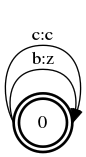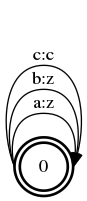# Introduction to Finite State Transducers

Finite state transducers are the older sibling of finite state automata. If you haven’t, take a few minutes to read through the introduction to FSA. An FSA only has one tape, or feed of data: input. An FST has two: input and output. So, whenever you transition on an edge in the graph, you consume some input (possibly ε, the empty string, “”) and output something (possibly ε). Edges are now labeled `i:o`, where `i` is the input for the edge and `o` is the output. If the edge has a weight, the edge will be labeled `i:o/w`, where w is the weight.

### OpenFST

Just like in the introduction to FSA, the figures in this post are made with OpenFST, an open source tool built by Google and NYU to manipulate finite state transducers. You can specify an FST to use in OpenFST with the following format:

`<source> <destination> <input> <ouput> [<weight>]`

Where `source` & `destination` are integer IDs of states and `weight` is optional. See the OpenFST docs.

### Example: Replace all `a`s with `b`s

Let’s say, for whatever reason, you have a thing against the letter `a`. Every `a` in your input should be replaced with a `b`. For simplicity, let’s assume that our input alphabet is `{a, b, c}`. Here’s an FST that does the trick:

``````\$ fstcompile --isymbols=<ascii.syms --osymbols=ascii.syms <a href="">ab.fst.txt</a> > ab.fst
\$ fstdraw --isymbols=ascii.syms --osymbols=ascii.syms ab.fst | dot -Tpng -Gsize=6,3 -Eheadport=e -Etailport=w > ab.png``````### Example: Capitalize every character

You can capitalize every character of the input by mapping lowercase letters to their uppercase version and mapping uppercase letters to themselves.

``````\$ fstcompile --isymbols=ascii.syms --osymbols=ascii.syms uppercase.fst.txt > uppercase.fst
\$ fstdraw --isymbols=ascii.syms --osymbols=ascii.syms uppercase.fst | dot -Tpng -Gsize=6,3 -Eheadport=e -Etailport=w > uppercase.png``````### Composition

The real power of finite state transducers comes from composition. When you compose two transducers, you feed the output of one into the input of the other. We usually use ○ to indicate composition of two transducers. So `A = B ○ C`, takes the input from the user, feeds it to `B`, takes the output from `B` and feeds it to `C`, then the result is the output from `C`. So, for example, if `A` has two states with one edge `a:b`, and `B` has two states with one edge `b:c`, then `C` will have two states with one edge `a:c`.

Let’s try composing the “replace every `a` with `b`” FST from above with an FST that turns `b`s into `z`s. We’ll start with an FST which replaces all `b`s with `z`s:

``\$ fstcompile --isymbols=ascii.syms --osymbols=ascii.syms bc.fst.txt > bc.fst``Then compose our previous FST with this one:

``fstcompose ab.fst bc.fst > abc.fst``With this transducer, if we input `abcab`, the output will be `zzczz`.

By tying multiple transducers together, you can come up with powerful pipelines – capable of doing speech recognition, case restoration, spelling correction, and more. For example, you could have one FST that can insert vowels into the input words (with some cost) and another FST that gives a weight to every word (more popular words get a lower cost). When you compose them, you’ll be left with an FST that can restore vowels in a sentence like, “Ths sntnc hd vwls rmvd” with high accuracy.

In the next post, we’ll create an FST to compute the Levenshtein edit distance of two strings.